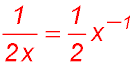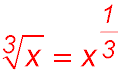Dr. J's Maths.com
Where the techniques of Maths
are explained in simple terms.

Algebra - Indices and Powers.
Main page.

Remember that we have the six basic strategies for manipulating indices:

 1 When multiplying terms with the same base, add the indices. When dividing terms with the same base, subtract the indices. d a × d b = d a+b. 2 When applying an index to a index, multiply the indices (and think - you have b groups of d a powers). (d a) b = d ab 3 When raising any base to an exponent of 1, the result is just the original base. x 1 = x 4 When raising any base to an exponent of 0 (zero), the result is always 1. x 0 = 1 5 Negative indices are used when moving terms between the numerator and denominator - or vice versa.6 When reformatting a radical of a number, use a fractional index.Introduction and basic questions.Most relevant to Junior School and Standard Maths courses in the Senior School. Basic concepts of indices - Test Yourself 1. Basic concepts of indices - Test Yourself 1 - Solutions. Basic concepts (slightly harder - Test Yourself 2. Basic concepts (slightly harder) - Test Yourself 2 - Solutions. More advanced questions.Most relevant to Maths courses at the top of the Junior school and to Advanced Maths courses in the Senior School. Advanced concepts of indices and problems - Test Yourself 3. Advanced concepts of indices and problems - Test Yourself 3 - Solutions.